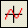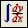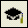Each button in the Math toolbar opens another toolbar of operators or symbols. You can insert many operators, Greek letters, and plots by clicking these buttons:Calculator: This button you can use for Arithmetic Operators.Graph: This button will give you 2 and 3 dimensional plot types as well as many graph tools.Matrix: This button will give you matrix and vector operators.Evaluation: This button gives you equal signs for evaluation and definition.Calculus: This  button gives you many calculus functions such as: derivatives, integrals, limits, and iterated sums and products.Boolean: This button will give you comparative and logical operators for Boolean expression.Programming: This button will set you up with different programming constructs.Greek: This button will give you Greek letters, which can be used as variables.Symbolic: This button gives you symbolic keywords and modifiers.   Referenced from: scrib.com